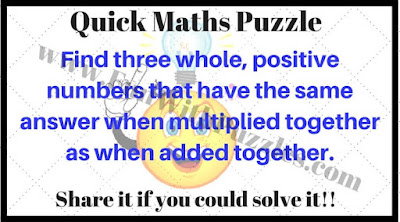For the last many days, we have been posting the different types of puzzles. These puzzle types include Logical Puzzles in which one has to logical solve some of the given mathematical equations and then solve the last equations. There are many different types of Maths Riddles in which one has to tell the missing number in the group of given numbers. Also, there are many different types of Picture Puzzles. Some of the picture puzzles are finding the Odd one Out among the given set of pictures and some of the Picture Puzzles are finding the hidden animals in the given pictures. Today brain teasers are different from all brain teasers.
These brain teasers are a mix of mathematical puzzles, logical puzzles, and out of box thinking puzzles. However, these brain teasers are not very tough and can be solved quickly. Try to solve these puzzles as quickly as possible.
Do post your timings to solve these puzzles in the comments section. Answers to these quick brain teasers are given at the end of these puzzle images.1. Quick Maths Puzzle Question2. Quick Maths Riddle3. Quick Thinking brain teaser4. Quick Thinking Puzzle Question5. Quick Thinking Tricky Brain Teaser